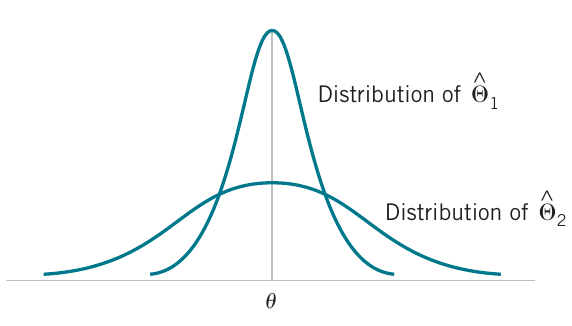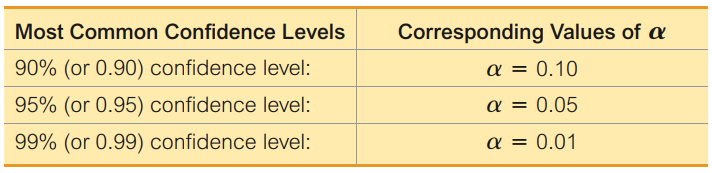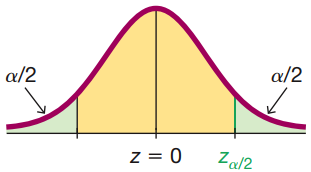# Point estimation and confidence intervals

04/07/2021

## Instructions:

Use the left and right arrow keys to navigate the presentation forward and backward respectively. You can also use the arrows at the bottom right of the screen to navigate with a mouse.

FAIR USE ACT DISCLAIMER:
This site is for educational purposes only. This website may contain copyrighted material, the use of which has not been specifically authorized by the copyright holders. The material is made available on this website as a way to advance teaching, and copyright-protected materials are used to the extent necessary to make this class function in a distance learning environment. The Fair Use Copyright Disclaimer is under section 107 of the Copyright Act of 1976, allowance is made for “fair use” for purposes such as criticism, comment, news reporting, teaching, scholarship, education and research.

## Outline

• The following topics will be covered in this lecture:

• General concepts in point estimation
• Bias of estimators
• Variance of estimators
• Standard Error
• An introduction to confidence intervals

## General concepts of point estimation

• Recall, any function of a random sample, i.e., any statistic, is modeled as a random variable.

• If $$h$$ is a general function used to compute some statistic, we thus define

$\hat{\Theta} = h(X_1, \cdots, X_n)$

to be a random variable that will depend on the particular realizations of $$X_1,\cdots, X_n$$.

• We call the probability distribution of a statistic a sampling distribution.

Sampling Distribution
The probability distribution of a statistic is called a sampling distribution.
• The sample mean

$\hat{\Theta} = \overline{X} = h(X_1, \cdots, X_n)= \frac{1}{n}\sum_{i=1}^n X_i$

is now one example for which we have a model of the sampling distribution.

• Specifically, the central limit theorem says that the sampling distribution of the sample mean is $$\overline{X} \sim N\left(\mu, \frac{\sigma^2}{n}\right)$$ when $$X$$ is normal, or if $$n$$ is sufficiently large.

### General concepts of point estimation continued

• Recall, we had a special name for $$\hat{\Theta}$$ in relation to the true parameter value $$\theta$$:
• Point estimators
A point estimate of some population parameter $$\theta$$ is a single numerical value $$\hat{\theta}$$ of a statistic $$\hat{\Theta}$$. This is a particular realization of the random variable $$\hat{\Theta}$$, viewed as a random variable; $$\hat{\Theta}$$ is called the point estimator.
• We want an estimator to be “close” in some sense to the true value of the unknown parameter, but we know that it happens to be a random variable.

• In this way, we need to describe how close this estimator is to the true value in a probabilistic sense.

• As we have seen before, there are important parameters that describe a probability distribution or a data set:

1. the “center” of the data / distribution; and
2. the “spread” of the data / distribution.
• The central limit theorem actually provided both of these (and the sampling distribution) for the sample mean:

1. the “center” of the distribution for $$\hat{\Theta}=\overline{X}$$ was given by $$\mu$$, the true population mean;
2. the “spread” of the distribution for $$\hat{\Theta}=\overline{X}$$ was given by $$\frac{\sigma}{\sqrt{n}}$$, the standard deviation of the population, divided by the square-root of the sample size.
• The two above parameters thus give us a means of describing “how close” the sample mean $$\overline{X}$$ tends to be to the population mean $$\mu$$ in a probabilistic sense.

### Bias of estimators

• The notion of the “center” of the sampling distribution can be useful as a general criteria for estimators.

• Formally, we say that $$\hat{\Theta}$$ is an unbiased estimator of $$\theta$$ if the expected value of $$\hat{\theta}$$ is equal to $$\theta$$.

• This is equivalent to saying that the mean of the probability distribution of $$\hat{\Theta}$$ (or the mean of the sampling distribution of $$\hat{\Theta}$$) is equal to $$\theta$$.

Bias of an Estimator
The point estimator $$\hat{\Theta}$$ is an unbiased estimator for the parameter $$\theta$$ if $\mathbb{E}\left[\hat{\Theta}\right] = \theta$ If the estimator is not unbiased, then the difference $\mathbb{E}\left[\hat{\Theta}\right] - \theta$ is called the bias of the estimator $$\hat{\Theta}$$. When an estimator is unbiased, the bias is zero; that is, \begin{align} \mathbb{E}\left[\hat{\Theta}\right] - \theta &= \theta - \theta \\ &=0 \end{align}
• If we consider the expected value to represent the average value over infinite replications;

• the above says that “over infinite replications of a random sample of size $$n$$, the average value of the point estimator $$\hat{\Theta}$$ will equal the true population parameter $$\theta$$”.
• A particular realization of $$\hat{\Theta}$$ will generally not equal the true value $$\theta$$.

• However, replications of the experiment will give a good approximation of the true value $$\theta$$.

## Variance of estimators

• We use the bias as discussed already to measure the center of a sampling distribution
• An unbiased estimator will have a distribution centered at the true population parameter.
• Yet suppose we have two estimators of the same parameter $$\theta$$, which we will denote $$\hat{\Theta}_1$$ and $$\hat{\Theta}_2$$ respectively.
• It is possible that they are both unbiased (the sampling distributions have the same center), yet they have different spread.
• That is to say, one estimator might tend to vary more than the other.Courtesy of Montgomery & Runger, Applied Statistics and Probability for Engineers, 7th edition

• The spread is a critical measure of how much variation is encountered with respect to resampling.
• We might describe the two concepts with an estimator as follows:
• Accuracy of an estimator - this is represented by the estimator being unbiased, so that we expect it to give an accurate result on average.
• Precision of an estimator - this is represented by the estimator having a small spread, so that the estimates don’t differ wildly from sample to sample.
• It is possible, in general, for an estimator to be either, both or neither of the above.
• We are often interested, thus, in unbiased estimators with a minimum variance as a first choice.
• In some situations biased estimators will actually be preferred, though a general discussion of the tradoffs is beyond our scope.

### Variance of estimators continued

• As a formal definition, we will introduce the following idea:
• Minimum Variance Unbiased Estimator
If we consider all unbiased estimators of $$\theta$$, the one with the smallest variance is called the minimum variance unbiased estimator (MVUE).
• The practical interpretation again is demonsrated by the last figure:Courtesy of Montgomery & Runger, Applied Statistics and Probability for Engineers, 7th edition

• Suppose that $$\hat{\Theta}_1$$ is the MVUE, and $$\hat{\Theta}_2$$ is any other unbiased estimator.
• Then, $\mathrm{var}\left(\hat{\Theta}_1\right) \leq \mathrm{var}\left(\hat{\Theta}_2\right).$
• Practically speaking, the MVUE is the most precise unbiased estimator, as its value changes the least with respect to resampling.
• An important example of a MVUE is actually the sample mean.
• If $$X_1, X_2 , \cdots , X_n$$ is a random sample of size $$n$$ from a normal distribution with mean $$\mu$$ and variance $$\sigma^2$$, the sample mean $$\overline{X}$$ is the MVUE for $$\mu$$.
• Again, other choices exist to estimate $$\mu$$, but among all unbiased estimators, the sample mean is the most precise.
• For non-normal distributions, however, a better choice might be, e.g., a biased estimator.

### Standard error of an estimator

• As noted before, the variance is a “natural” measure of spread mathematically for theoretical reasons, but it is in the units squared of the original units.

• For this reason, when we talk about the spread of an estimator's sampling distribution, we typically discuss the standard error.

The standard error Let $$\hat{\Theta}$$ be an estimator of $$\theta$$. The standard error error of $$\hat{\Theta}$$ is its standard deviation given by $\sigma_\hat{\Theta} = \sqrt{\mathrm{var}\left(\hat{\Theta}\right)}.$ If the standard error involves unknown parameters that can be estimated, substitution of those values into the equation above produces an estimated standard error denoted $$\hat{\sigma}_\hat{\Theta}$$. It is also common to write the standard error as $$\mathrm{SE}\left(\hat{\Theta}\right)$$.
• Q: can anyone recall what the standard error is of the sample mean? That is, what is the standard deviation of the sampling distribution (for a normal sample or $$n$$ large)?

• A: the central limit theorem states that $$\overline{X}$$ follows (exactly for a normal sample or $$n$$ large, approximately) a sampling distribution

$\overline{X}\sim N\left(\mu, \frac{\sigma^2}{n}\right).$

• Therefore, the standard error of the sample mean is precisely,

$\sigma_{\overline{X}} = \frac{\sigma}{\sqrt{n}}.$

### Standard error of an estimator

• As was discussed before, there are times that we may not know all the parameters that describe the standard error.

• For example, suppose we draw $$X_1, \cdots, X_n$$ from a normal population, for which we know neither the mean nor the variance.

• Let the unknown and unobservable theoretical parameters be denoted $$\mu$$ and $$\sigma$$ as usual.

• The sample mean has the sampling distribution,

$\overline{X} \sim N\left( \mu, \frac{\sigma^2}{n}\right),$

and therefore standard error $$\sigma_{\overline{X}} = \frac{\sigma}{\sqrt{n}}$$.

• However, we stated that $$\sigma$$ itself is unknown.

• In this case, we will estimate the standard error as

$\hat{\sigma}_\overline{X} = \frac{s}{\sqrt{n}}$ with the sample standard deviation $$s$$.

• This is what is meant to estimate the standard error.

• This particular example will be extremely important for confidence intervals.

### Standard error of an estimator – example

• An article in the Journal of Heat Transfer (Trans. ASME, Sec. C, 96, 1974, p. 59) described a new method of measuring the thermal conductivity of Armco iron.

• Using a temperature of $$100^\circ$$ F and a power input of 550 watts, the following 10 measurements of thermal conductivity (in Btu/hr-ft-∘ F) were obtained:

$41.60, 41.48, 42.34, 41.95, 41.86, 42.18, 41.72, 42.26, 41.81, 42.04$

• A point estimate of the mean thermal conductivity at $$100^\circ$$ F and 550 watts is the sample mean or

$\overline{x} = 41.924$

• The standard error of the sample mean is $$\sigma_\overline{X}=\frac{\sigma}{\sqrt{n}}$$;

• however, $$\sigma$$ is unknown so that we estimate it by the sample standard deviation $$s = 0.284$$ to obtain

$\hat{\sigma}_\overline{X} = \frac{s}{\sqrt{n}}= \frac{0.284}{\sqrt{10}} \approx 0.0898$

• Notice that the standard error is about 0.2 percent of the sample mean, implying that we have obtained a relatively precise point estimate of thermal conductivity.

### Standard error of an estimator – example

• Assume that thermal conductivity is normally distributed, then two times the standard error is

$2\hat{\sigma}_\overline{X} = 2(0.0898) = 0.1796.$

• The empirical rule says that about 95% of realizations of the sample mean lie within two standard deviations of the true mean $$\mu$$.

• Therefore, we are highly confident that the true mean thermal conductivity is within the interval 41.924 ± 0.1796 or between $$[41.744 , 42.104]$$.

• We will now formalize this logic into confidence intervals.

## Introduction to confidence intervals

• Engineers are often involved in estimating parameters.

• For example, there is an ASTM Standard E23 that defines a technique called the Charpy V-notch method for notched bar impact testing of metallic materials.

• The impact energy is often used to determine whether the material experiences a ductile-to-brittle transition as the temperature decreases.

• Suppose that we have tested a sample of $$10$$ specimens of a particular material with this procedure. We know that we can use the sample average $$\overline{X}$$ to estimate the true mean impact energy $$\mu$$.

• However, we also know that the true mean impact energy is unlikely to be exactly equal to your estimate due to sampling error.

• Reporting the results of your test as a single number is unappealing because $$\overline{X}$$ may be an accurate estimator, but it doesn't tell us how precise the estimate is.

• Rather, measures of the spread of the sampling distribution, e.g., the standard error tell us how precise the estimate is.
• A way to quantify our uncertainty is to report the estimate in terms of a range of plausible values called a confidence interval.

### Introduction to confidence intervals – continued

• Suppose $$\hat{\Theta}$$ is an unbiased estimator for the speed of light $$\theta$$.
• We are assuming that $$\theta$$ is a deterministic but unknown constant.

• For sake of example, also suppose that $$\hat{\Theta}$$ has standard deviation $$\sigma_\hat{\Theta}$$ = 100 km/sec.
• Recall Chebyshev’s inequality,

\begin{align} P\left(\vert \hat{\Theta} - \theta \vert \geq k \sigma_\hat{\Theta}\right) \leq \frac{1}{k^2} \end{align}

• We find

\begin{align} P\left(\vert \hat{\Theta} - \theta \vert < 2 \sigma_\hat{\Theta}\right) > \frac{3}{4} \end{align}

• This tells us that there is a probability of at least 75% that $$\hat{\Theta}$$ is within 200 km/sec of the speed of light $$\theta$$.
• Equivalently, $$\theta \in \left(\hat{\Theta}-200, \hat{\Theta}+200\right)$$ with probability 75%.
• Notice that $$\left(\hat{\Theta}-200, \hat{\Theta}+200\right)$$ is a random interval but we again assume that $$\theta$$ is a fixed constant.

### Introduction to confidence intervals – continued

• Now we will suppose the estimate $$\hat{\Theta}$$ gives us based on some data is $$\hat{\theta}=299852.4$$

• We can say that $$\theta \in (299652.4, 300 052.4)$$ with confidence 75%.

• Note that $$\theta$$ is an an unknown constant – it is either in the interval or not and there is nothing random about the above statement.
• Therefore, we cannot say that the probability of $$\theta \in (299652.4, 300 052.4)$$ is 75%, but we used information to guarantee that our procedure for estimation will work 75% of the time.
• Confidence intervals give us a systematic procedure as above to guarantee with a level of confidence that our plausible values for the parameter $$\theta$$ include the true value.

• In the remaining course, we will be concerned with dual questions:

1. With what confidence can we estimate a parameter $$\theta$$ as a range of plausible values? And
2. how unlikely would it be for $$\theta$$ to be outside of some range based on our observations?
• These two ideas are known as confidence intervals and hypothesis testing respectively.

### Introduction to confidence intervals – continued

• Suppose that $$X_1 , X_2, \cdots , X_n$$ is a random sample from a normal distribution with unknown mean $$\mu$$ and known variance $$\sigma^2$$.

• By the central limit theorem, we know that the sample mean $$\overline{X}$$ is distributed

$\overline{X} \sim N\left(\mu, \frac{\sigma^2}{n}\right)$

• We may standardize $$\overline{X}$$ by subtracting its mean and dividing by its standard deviation,

\begin{align} Z = \frac{\overline{X} - \mu}{\frac{\sigma}{\sqrt{n}}}. \end{align}

• The random variable $$Z$$ has a standard normal distribution.

• A confidence interval estimate for $$\mu$$ is an interval of the form

$l ≤ \mu ≤ u,$ where the end-points $$l$$ and $$u$$ are computed from the sample data.

• Because different samples will produce different values of $$l$$ and $$u$$, these end-points are values of random variables $$L$$ and $$U$$, respectively.

### Introduction to confidence intervals – continued

• Suppose that we can determine values of $$L$$ and $$U$$ such that the following probability statement is true:

$P\{L \leq \mu \leq U\} = 1 − \alpha$ where $$0 \leq \alpha \leq 1$$.

• There is a probability of $$1 − \alpha$$ of selecting a sample for which the CI will contain the true value of $$\mu$$.

• Once we have selected the sample, so that $$X_1 = x_1 , X_2 = x_2 , \cdots , X_n = x_n$$, and computed $$l$$ and $$u$$, the resulting confidence interval for $$\mu$$ is

$l \leq \mu \leq u.$

• The end-points or bounds $$l$$ and $$u$$ are called the lower- and upper-confidence limits (bounds), respectively, and $$1 − \alpha$$ is called the confidence coefficient (or level).

• Again,

$$l \leq \mu \leq u.$$ is a fixed numerical statement with nothing random about it, this is true or untrue.

• The goal then is to find the procedure that defines the random variables $$L,U$$ for which the procedure will succeed $$(1-\alpha)\times 100\%$$ of the time.

### Introduction to confidence intervals – continuedCourtesy of Mario Triola, Essentials of Statistics, 6th edition

• Let’s take an example confidence level of $$95\%$$ – this corresponds to a rate of failure of $$5\%$$ over infinitely many replications.
• Generally, we will write the confidence level as, $(1 - \alpha) \times 100\%$ so that we can associate this confidence level with its rate of failure $$\alpha$$.
• In our problem, because $$Z = \frac{\overline{X} - \mu }{\frac{\sigma}{\sqrt{n}}}$$ has a standard normal distribution, we may write \begin{align} P\left(-z_\frac{\alpha}{2} \leq \frac{\overline{X} - \mu }{\frac{\sigma}{\sqrt{n}}} \leq z_\frac{\alpha}{2}\right) = 1 - \alpha \end{align} where
• we want to find the critical value $$z_\frac{\alpha}{2}$$ for which:
• $$(1-\frac{\alpha}{2})\times 100\%$$ of the area under the normal density lies to the left of $$z_\frac{\alpha}{2}$$; and
• $$(1-\frac{\alpha}{2})\times 100\%$$ of the area under the normal density lies to the right of $$-z_\frac{\alpha}{2}$$.
• Put together, $$(1-\alpha)\times 100\%$$ of values lie within $$[-z_\frac{\alpha}{2},z_\frac{\alpha}{2}]$$ in the standard normal.